# How to use the SUMIF Function in Google Sheets (with Worksheet and Examples)

The Google Sheets sumif function is a conditional formula, similar to COUNTIF, that lets you find the sum of cells that meet certain criteria.

That is, we can conditionally sum cells only if they fall within a certain specified criteria (or within multiple criteria) such as ">cell reference" or if a certain cell reference matches a specified criteria such as "Pool Store" (we will use that as our first example).

So, let's dive into how to properly use the SUMIF function in Google Sheets to conditionally sum cells in a variety of different case scenarios. We will share multiple sumif function examples along the way.

You can access the SUMIF examples worksheet here at any time. You will need to select "make a copy to edit" if you want to edit the Google sheet with your own data and custom cell ranges and cell references.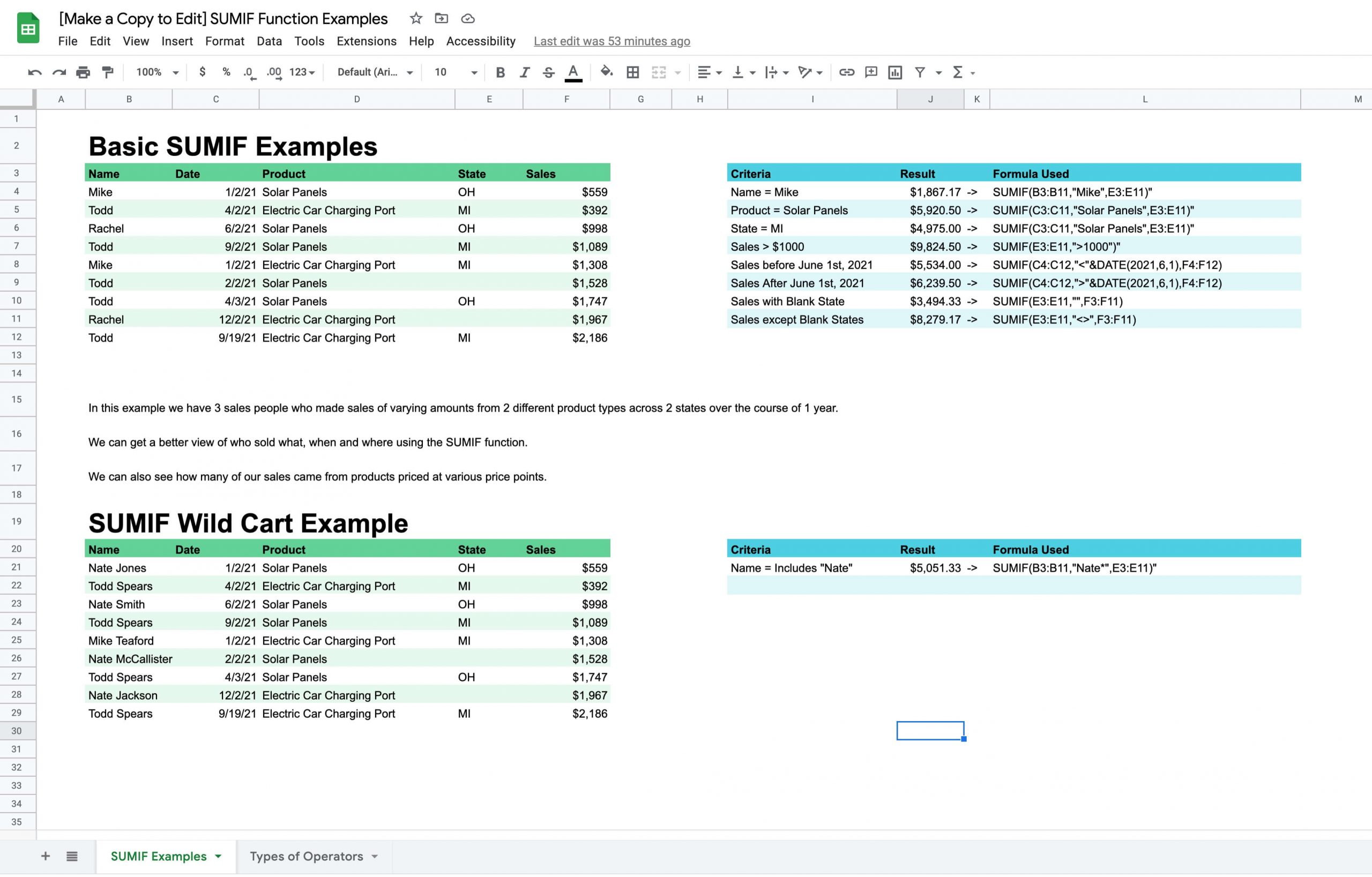Note: This process is the same on Microsoft Excel. To follow this formula you will of course need to understand the more basic Google sheets formula for =SUM functions, so be sure click the link here and read that article first if for some reason you don't know how to do that yet.

## SUMIF Real World Application Examples

Potential uses might include:

• You have a year of sales data. You want to find the sum of sales that happened after July 31st, 2021.
• You have 3 sales people, (John, Lisa and Tammy) and a list of 1,000 sales they made. You want to see the total value of sales that John made.
• You have a year of sales data. You want to find the sum of sales that were over \$1,000 each.

The SUMIF function is essentially an alternative to a basic filter and potential use cases can be found across nearly any field that has data that can be added.

## SUMIF Formula Explained

Here is what the general pattern for a SUMIF formula looks like.

=SUMIF(range,"criteria",sum_range)

With numbers, it might look something like this...

=SUMIF(B3:B11,"Mike",E3:E11)

A SUMIF formula is broken down into multiple parts.

We have 2 ranges, the range that must meet our criteria and then the sum range with includes the numerical data we will count in our sum if the criteria are met.

Between the two ranges is the criteria. This dictates which rows or columns we will count in our sum and which we will leave out.

## SUMIF Function with Keywords as Criteria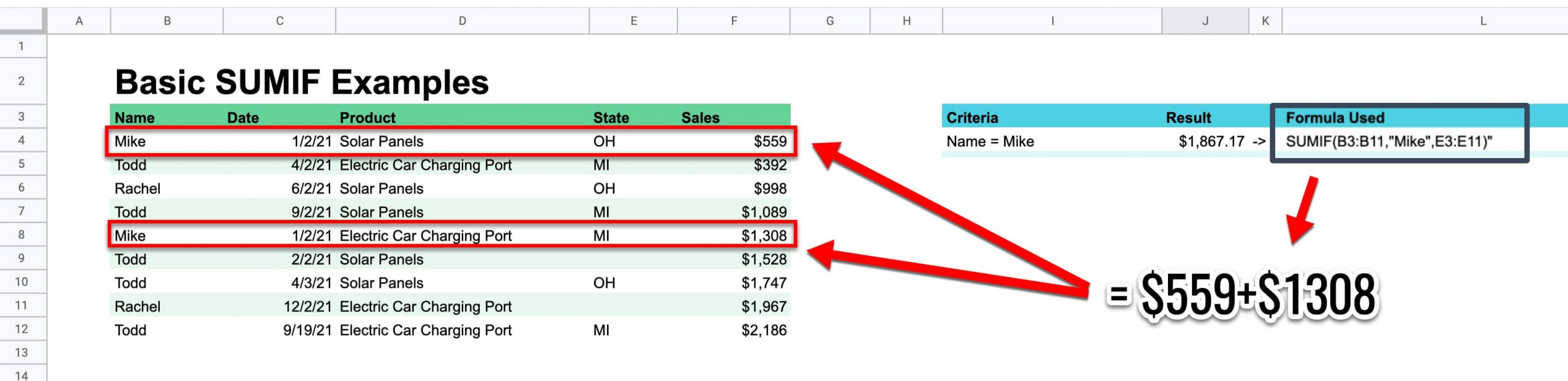First, I will show you how to look up conditional sums with respect to keywords. These will need to be exact matches in the given cell range to work.

Here is how to do it.

1. Type =SUMIF( to initiate the equation.
2. Declare the range from which you will be scanning. I want to look for all of the Pool Store Profits, So I will set the range to be D7 to D16.
3. In quotations, declare what keyword you want to look for. I want to know how much the pool made this month in sales from the Pool Store, so I will input “Pool Store”. The double quotation marks are critical to making this work.
4. Declare the sum range. For this , you will want to select the range of cells from the Profit column, meaning the range will be E7 to E16.

If done correctly, the equation should have added up every net profit declaration that came from the pool store this month.

## SUMIF Formula with Numerical Range as Criteria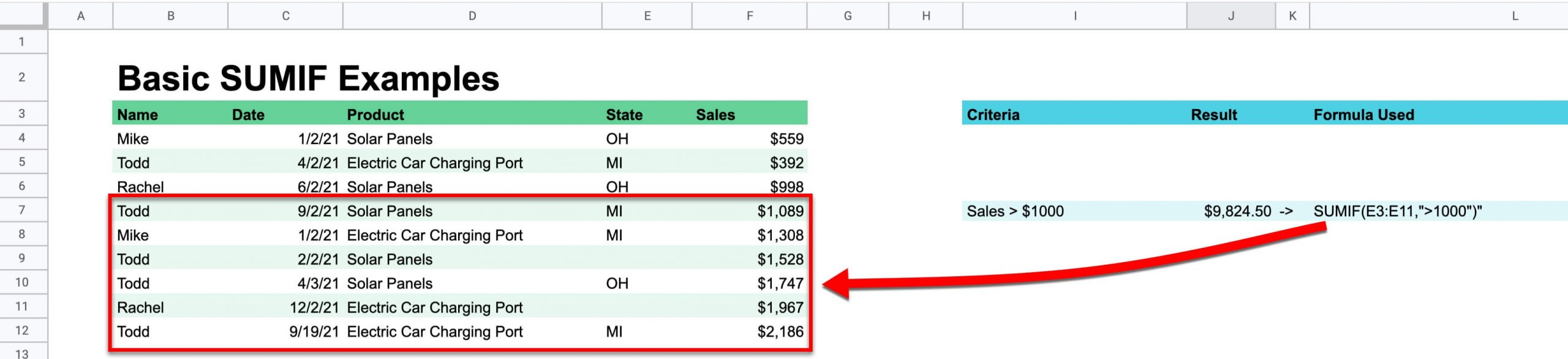You can also find sums within certain Margins. When you want to find the sum based on criteria of the sum column, it is a little easier because you only need to declare one range.

Note, we can also do the formula without the first range if we use a logical operator, a criteria and the sum_range. This is useful if we want to count only values that meet a criteria that involves their value.

For example, if we want to only count the cells that have values over \$100.

That formula pattern looks like this

=SUMIF(sum_range,"logicaloperator+criteria")

With data, it might look something like this...

=SUMIF(E3:E11,">1000")

Notice the logical operator in this variation of the SUMIF function. The logical operator will tell us how to compare values.It can be represented as less than (<) , greater than (>), less than or equal to (<=), greater than or equal to (>=), equal to (=) and not equal to (<>).

## SUMIF Formula with Date Function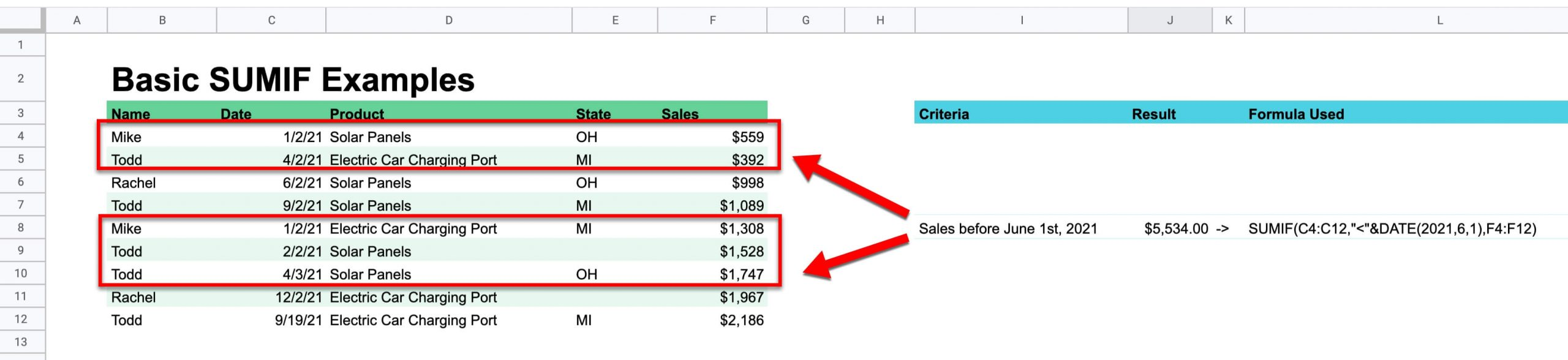We can sum the numbers based on date criteria by using what are called "comparison operators." To do this, the date must be provided in double quotations marks or with a proper date function such as =TODAY() to be understood by Google Sheets or Microsoft Excel.

We will use logical operators before the dates. If we want dates before a certain time, we use the less than operator and we use the greater than operator if we want a date after a certain time.

The SUMIF function has a lot of practical application when used with the date function. For example, if we have a report of transactions over a given 365 day period, we can find the sum of all sales that happened after June 1st, 2021.

Here is what that function would look like if the data was provided as follows below.

=SUMIF(C4:C12,">"&DATE(2021,6,1),F4:F12)

## SUMIF Formula with Blank Cell Reference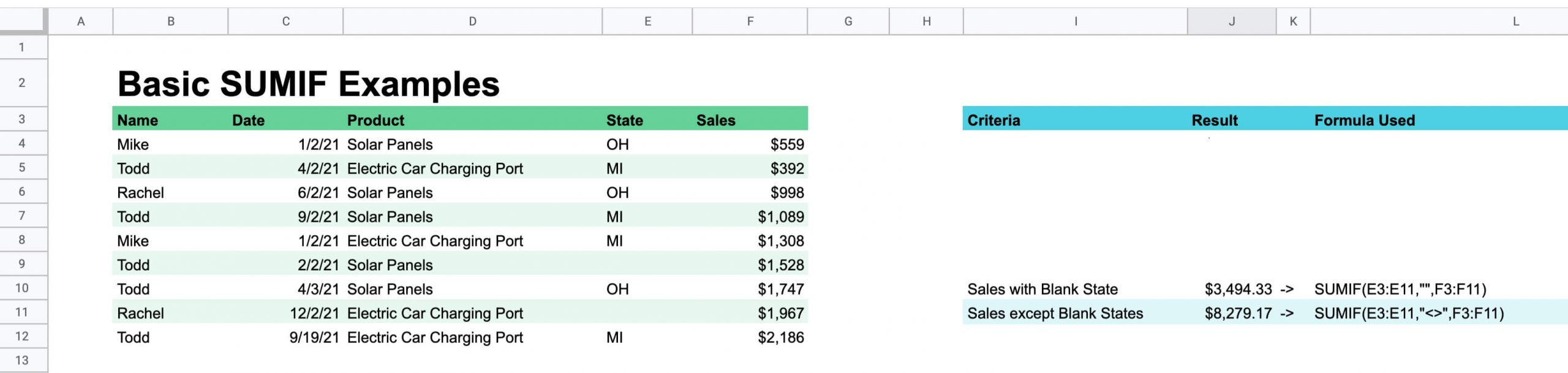There might be a time when you want to remove data that is incomplete or you want to count only data from ranges that have a certain cell left empty.

We can do this with the SUMIF formula like this.

If we want to add cells that are blank, the formula looks as follows.

=SUMIF(range,"",sum_range)

We simply replace the criteria with double quotation marks and nothing between.

If we want to remove from the sum ranges that have blanks, we would use the following formula.

=SUMIF(range,"<>",sum_range)

We simply replace the criteria with the "not equal" sign (<>).

## SUMIF Formula with WildcardIn excel and Google Sheets, we can use what is called a "wildcard."

Putting an asterisk in the criteria opens up the potential uses so the cells do not have to be exact matches.

For example, if I have 3 sales people named "Nate" but they all have last names and my cells are broken down by First and Last Names

That function would look like this.

=SUMIF(range,"Nate*",sum_range"

This is a pretty nonsensical example, but the concept can actually be quite useful if you keep your eye out for ways to apply it.

## IMPORTANT SUMIF NOTES

• SUMIFS requires a range, you can't substitute and you can't substitute an array.
• SUMIF can only support one condition.For multiple criteria, you will need to use the SUMIFS (plural) function.
• When you omit the sum_range, the cells in range will be summed.
• Double quotes are mandatory for text strings in criteria.
• Cell references in criteria however are not enclosed in quotes.

Have any questions about SUMIF functions? Let me know down in the comments!